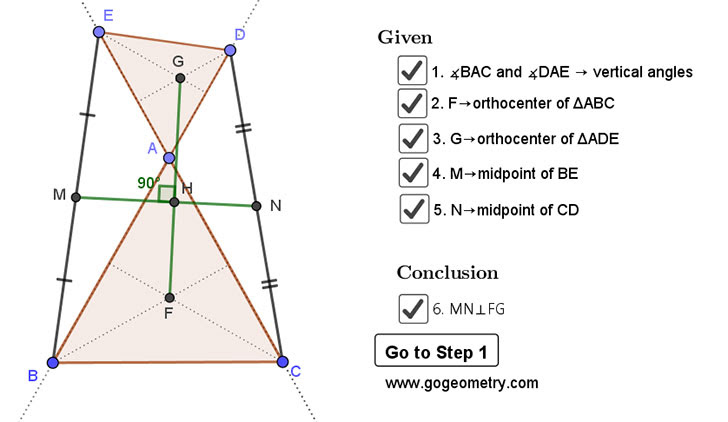## Sunday, March 8, 2020

### Geometry Problem 1459: Two Triangles, Orthocenter, Midpoint, Perpendicular

Interactive step-by-step animation using GeoGebra. Post your solution in the comment box below.
Level: Mathematics Education, High School, Honors Geometry, College.

Details: Click on the figure below.#### 1 comment:

1.Let circumradius of Tr.AED be R
Then AG=2R.cos(A)=(ED/sin(A)).cos(A)=ED/tan(A)
Similarly in Tr. ABC AF= BC/tan(A),
We have AG/AF = ED/BC.
Let midpoint of BD is J. Then MJ=ED/2 and NJ=BC/2.
MJ/NJ=ED/BC, also MJ and NJ are parallel to ED and BC respectively.
We get MJ/NJ=AG/AF, AG and AF are 90 degree clock wise rotation of MJ and NJ.
Hence Tr.MJN and Tr.GAF are similar, and GF is 90 degree clockwise rotation of MN.
Hence MN is perpendicular to FG.